# Arduino Voltmeter

Voltmeter using arduino amd LCD display.

IntermediateFull instructions provided8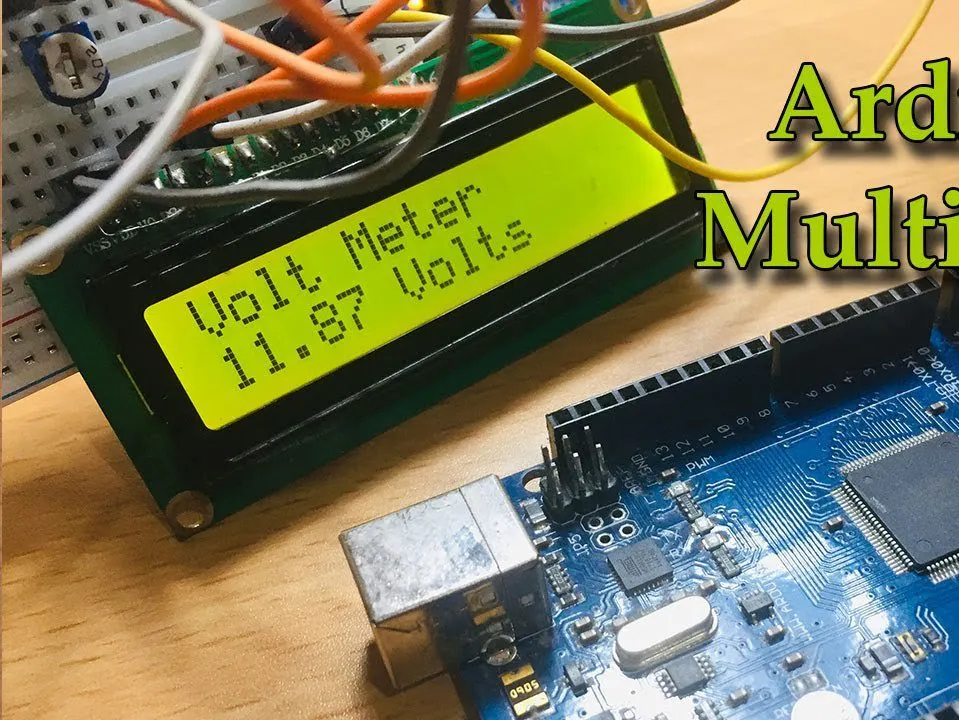## Things used in this project

### Hardware components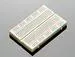×1Jumper wires (generic)
×1Resistor 10k ohm
×1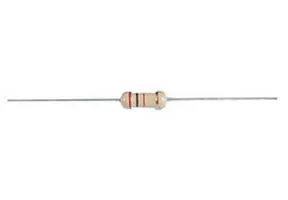Through Hole Resistor, 120 ohm
×1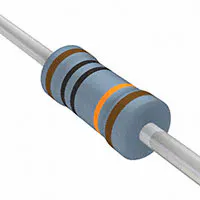Resistor 100k ohm
×1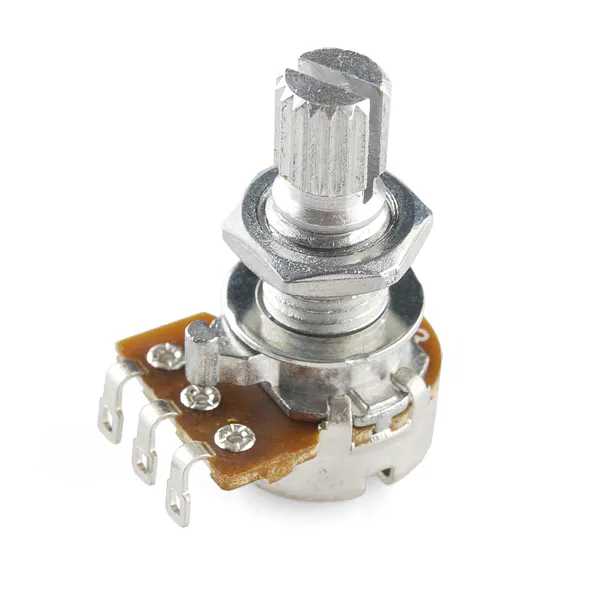Rotary potentiometer (generic)
×1Arduino UNO
×1

### Hand tools and fabrication machines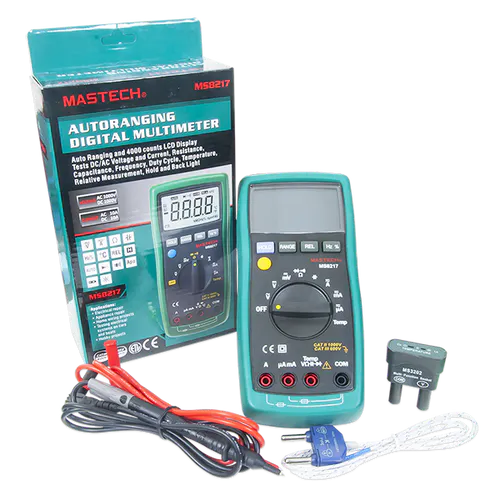Digilent Mastech MS8217 Autorange Digital MultimeterSoldering iron (generic)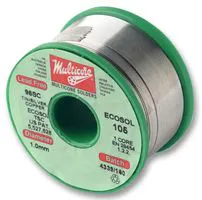## Schematics

### Circuit

There are two diagrams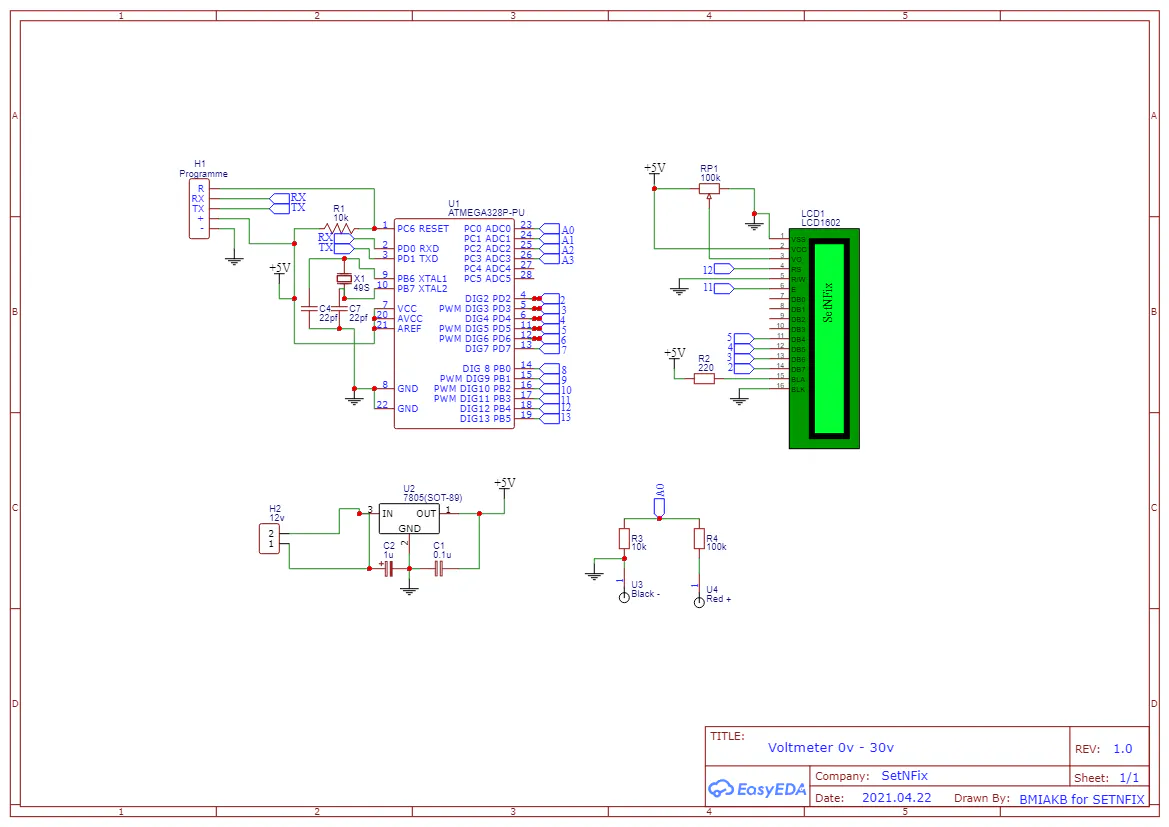## Code

### Arduino Voltmeter

Arduino
Arduino code for voltmeter
```#include <LiquidCrystal.h>

/*
LiquidCrystal Library - Hello World

Demonstrates the use a 16x2 LCD display.  The LiquidCrystal
library works with all LCD displays that are compatible with the
Hitachi HD44780 driver. There are many of them out there, and you
can usually tell them by the 16-pin interface.

This sketch prints "Hello World!" to the LCD
and shows the time.

The circuit:
* LCD RS pin to digital pin 12
* LCD Enable pin to digital pin 11
* LCD D4 pin to digital pin 5
* LCD D5 pin to digital pin 4
* LCD D6 pin to digital pin 3
* LCD D7 pin to digital pin 2
* LCD R/W pin to ground
* LCD VSS pin to ground
* LCD VCC pin to 5V
* 10K resistor:
* ends to +5V and ground
* wiper to LCD VO pin (pin 3)

Library originally added 18 Apr 2008
by David A. Mellis
library modified 5 Jul 2009
by Tom Igoe
modified 22 Nov 2010
by Tom Igoe
modified 7 Nov 2016

This example code is in the public domain.

http://www.arduino.cc/en/Tutorial/LiquidCrystalHelloWorld

Modified by : BMIAK Basnayaka for SETNFIX
Date : 22/04/2021
http://setnfix.com

*/

// include the library code:
#include <LiquidCrystal.h>

// initialize the library by associating any needed LCD interface pin
// with the arduino pin number it is connected to
const int rs = 12, en = 11, d4 = 5, d5 = 4, d6 = 3, d7 = 2;
LiquidCrystal lcd(rs, en, d4, d5, d6, d7);
float vout = 0.0;
float vin = 0.0;
float R1 = 100000.0; // resistance of R1 (100K) -see text!
float R2 = 10000.0; // resistance of R2 (10K) - see text!
int value = 0;

void setup() {
// set up the LCD's number of columns and rows:
lcd.begin(16, 2);
// Print a message to the LCD.

lcd.print("SetNFix");
Serial.begin(9600);
delay(5000);
}

void loop() {

vout = (value * 5.0) / 1024.0; // see text
vin = vout / (R2/(R1+R2));
if (vin<0.09) {
vin=0.0;//statement to quash undesired reading !
}
// set the cursor to column 0, line 1
// (note: line 1 is the second row, since counting begins with 0):
lcd.setCursor(0, 0);
lcd.print("Volt Meter");
lcd.setCursor(0, 1);
// print the number of seconds since reset:
unsigned int len = sizeof(vin);
len = len +2;

lcd.print(vin);
lcd.print(" ");
//lcd.setCursor(len, 1);
lcd.print("Volts");
lcd.print(" ");
}
```

## Credits

### SetNFix

14 projects • 16 followers
I am an accountant in profession but, I would more prefer to work with electronics based innovations.